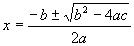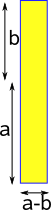A quadratic function has an x² term, its general form is:

a x² + b x + c = 0

A quadratic equation is one in which the unknown is in the second degree. Usually a quadratic has two solutions or roots:

eg if:

x² = 36

x = √36

x = +6 or -6

can be written as:

x = ± 6

## Methods of solution

1. by factors
2. by completing the square
3. by formulae
4. by graph

### Factorising

The first step in factorising is to find the highest common factor in expressional terms

4 l² x² - 2 l x3

2 l x² (2 l - x)

### Factors of a² - b²

a² - b² = (a+b)(a-b)

This can be used to factorise the difference of any two squares

## Factorising

solve the equation

3 x² = 2 x +5

3 x² - 2 x - 5 = 0

(3x - 5)(x+1) =0

so either (3x - 5) =0 or (x+1) =0

so either x = 3/5 or x=-1

## completing the square

The basis of this method is the forming of a perfect square, examples of perfect squares are:

• (x+1)² = x² + 2 x + 1
• (x-4)² = x² - 8 x + 16

Consider the equation x² + 10x

To form a perfect square a number is added which is the square of 1/2 the coefiant of x i.e.:

x² + 10x + (10/2)²

= x² + 10x +25

= (x+5) ²

Another example

6 x² + 11 x = 10

first step: divide both sides by the coeficient of x²(ie 6)

6 x² /6 + 11/6 x = 10/6

second step: make a perfect square by adding to both sides the square of 1/2 the coeficient of x ie (11/12) ²

x² + 11/6 x + (11/12) ² = 10/6 + (11/12) ²

(x + 11/12) ² = 136/144

(x + 11/12) =± √(136/144)

x = - 11/12 ± 19/12

x = -30/12 or 8/12

x = -2.5 or 0.667

## Solution by formula

if

a x² + b x + c = 0

 then x= -b ± √(b² - 4ac ) 2a

This formula can be proved by following the completing the square method with algebraic constants a,b and c instead of actual numbers:

a x² + b x + c = 0

a x² + b x = -c

x² + b/a x = -c/a

x² + b/a x + (b/2a)² = -c/a + (b/2a)²

(x + b/2a)² = -c/a + b²/4a²

x + b/2a = ± √(-c/a + b²/4a²)### Types of solution

The solution depends whether:

 b² - 4ac > 0 two real solutions b² - 4ac = 0 one solution b² - 4ac < 0 complex number solutions

Not every quadratic equation has a solution for real numbers, however there is an algebraic system which has a solution for every quadratic equation, these are the complex numbers.

Consider the following example:

x² - 6x + 10 = 0

from the formula we get:

x = ( 6 ± √(-4) )/2

Since there is no real root to √-4 it is expressed

√(-4) = √2*(-1)

√(-1) is called 'i'

therefore √(-4) = ±2i

therfore the solution to the problem is:

x = 3 ± i

## Using Symmetry

a x² + b x + c = 0

we can use the equation:

(x - x1)*(x - x2) = 0

which has solutions at:

x = x1 and x = x2

where:

x1 + x2 = -b/a

x1 * x2 = c/a

The equation (x - x1)*(x - x2) = 0 is much more symmetrical in that x1and x2can be swapped with each other without changing the result.

solutions are = ((x1 + x2) ± (x1 - x2))/2

where:

• x1 + x2 = -b/a
• x1 - x2 = √((x1 + x2)² - 4(x1 * x2)) = √((b/a)² - 4(c/a))
• x1 * x2 = c/a

### Quadratic Equations in Two Variables

We can represent a general quadratic equation in two variables as:

A x² + B xy + C y²+ D x + E y + F = 0

In the same way that the quadratic equation in one variable has different classes of solution (real, complex, etc.) so our quadratic equation in two variables has different types of solution.

circle x² + y² = r²
ellipse
 x² + y² =±1 a² b²
parabola y² = 4 a x
hyperbola
 x² - y² =±1 a² b²

These types can all be visualised as conic sections as shown on this page.

## Useful Identities

Here are some results which may help to speed up our calculations.

### Difference of 2 squares

 a²-b²= (a+b)(a-b)## Program

There are a number of open source programs that can solve polynomial equations. I have used Axiom, how to install Axiom here.

To get a numeric solution for a given equation we can use complexSolve as shown here:

complexSolve(3*x^2+4*x+5 = 0,1.e-10)

I have put user input in red:

 (1) -> complexSolve(3*x^2+4*x+5 = 0,1.e-10) (1) [x= - 0.6666666666 6666666667 - 1.1055415968 003217131 %i, x= - 0.6666666666 6666666667 + 1.1055415968 003217131 %i] Type: List Equation Polynomial Complex Float (2) ->

Or we can find a formula for, say, a quadratic equation using radicalSolve as shown here:

 ```(3) -> radicalSolve(a*x^2 + b*x + c = 0,x) ``` ``` +-----------+ +-----------+ | 2 | 2 - \|- 4a c + b - b \|- 4a c + b - b (3) [x= --------------------,x= ------------------] 2a 2a Type: List Equation Expression Integer ```

## Code

Here is a Java function to return the quadratic roots

```/**
code to solve quadratic equation ax^2 + bx + c =0
result we be returned in resultReal,resultImaginary,resultReal and resultImaginary
expects arrays to be setup before being called, like this:
double[] resultReal= new double;
double[] resultImaginary = new double;
*/
public void quadratic(double a, double b, double c, double[] resultReal , double[] resultImaginary) {
if (Math.abs(a) < 0.000001) { // if a=0 equation is linear
// handle seperately to avoid divide by zero
if (Math.abs(b) < 0.000001)
resultReal = 0; // x is undetermined
else
resultReal = -c/b;
resultReal =resultImaginary = resultImaginary = 0;
return;
}
double inner = b*b - 4*a*c;
if (inner < 0) {
// roots are complex
double s = Math.sqrt(-inner);
resultReal = resultReal = -b/(2*a);
resultImaginary = s/(2*a);
resultImaginary = -resultImaginary;
return;
}
double s = Math.sqrt(inner);
resultImaginary = resultImaginary = 0;
resultReal = (-b+s)/(2*a);
resultReal = (-b-s)/(2*a);
return;
} ```

 metadata block see also: Correspondence about this page Book Shop - Further reading. Where I can, I have put links to Amazon for books that are relevant to the subject, click on the appropriate country flag to get more details of the book or to buy it from them.Mathematics for 3D game Programming - Includes introduction to Vectors, Matrices, Transforms and Trigonometry. (But no euler angles or quaternions). Also includes ray tracing and some linear & rotational physics also collision detection (but not collision response). Other Math Books Specific to this page here:

This site may have errors. Don't use for critical systems.